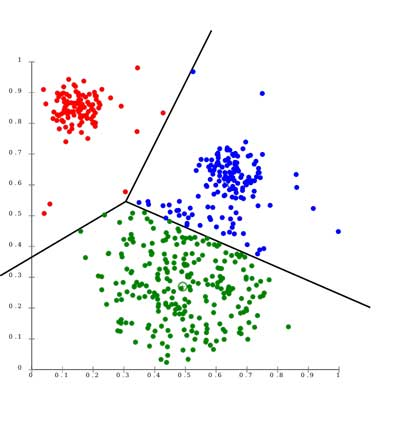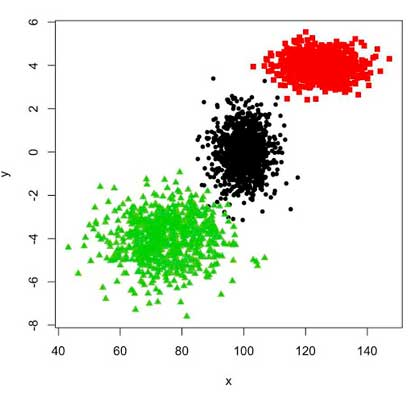## K-means Clustering Service

The k-means clustering algorithm is an iterative clustering analysis algorithm. The steps are to divide the data into K groups in advance, then randomly select K objects as the initial clustering centers, and then calculate The distance between each object and each seed cluster center, and each object is assigned to the cluster center closest to it. The cluster centers and the objects assigned to them represent a cluster. Each time a sample is allocated, the cluster center of the cluster will be recalculated based on the existing objects in the cluster. This process will be repeated until a certain termination condition is met. The termination condition can be that no (or minimum number) of objects are reassigned to different clusters, no (or minimum number) of cluster centers change again, and the sum of squared errors is locally minimum.

### AlgorithmFirst randomly select K objects as the initial cluster centers. Then calculate the distance between each object and each seed cluster center, and assign each object to the cluster center closest to it. The cluster centers and the objects assigned to them represent a cluster. Once all objects are assigned, the cluster center of each cluster will be recalculated based on the existing objects in the cluster. This process will be repeated until a certain termination condition is met. The termination condition can be any of the following:

1) No (or minimum number) of objects are reassigned to different clusters.
2) No (or minimum number) of cluster centers change again.
3) The sum of squared errors is locally minimum.

Fake code

Choose k points as the initial centroid.

repeat Assign each point to the nearest centroid to form k clusters Recalculate the centroid of each cluster until the centroid does not change.

### Our solutions

MedAI can provide you with professional K-means clustering service:The goal of K-means is to divide the data point into k clusters, find the center of each cluster, and minimize the function. Among them is the center of the i-th cluster. The above formula requires each data point to be as close as possible to the center of the cluster to which they belong. In order to get the center of each cluster, K-means performs two steps iteratively. First, the positions of k centers are randomly given, and then each data point is classified to the center closest to it, so that we construct k clusters. However, the positions of these k centers are obviously incorrect, so the centers must be transferred to the average position of the data points inside the cluster. In fact, it is calculation. When the classification of each data point is determined, the above function takes the position of the extreme value, and then constructs new k clusters again. During this process, the position of the center point is constantly changing, and the constructed cluster is also changing (see the animation here). After multiple iterations, these k centers will eventually converge and no longer move.

### Services items

Project name K-means Clustering Service
• Fast, robust and easier to use and understand.
• Relatively efficient: with a large number of variables, k-means may be computationally faster than hierarchical clustering method (if k is small).
• K-means may create tighter clusters than hierarchical clustering, particularly if the clusters are globular.
• Gives best result when data set are distinct or well separated from each other.
Cycle Depends on the time you need to simulate and the time required for the system to reach equilibrium.
Product delivery mode The simulation results provide you with the raw data and analysis results of molecular dynamics.
Price Inquiry

MedAI provides corresponding professional K-means clustering service. Our K-means clustering service has proven to be very useful for understanding the biochemical basis of physiological events at different stages of drug development (even in different fields such as materials science). The MedAI team has worked in this field for more than a decade and published his findings in top scientific journals. If you need network analysis services, please feel free to contact us.

## Online Inquiry